# ML Wiki

## Linear Hashing

• another dynamic hashing schema
• but without a directory that doubles in size as in Extensible Hashing

### Variables we use

• $b$ - length of bit-string that Hash Function outputs (typically 64)
• $i$ - number of bits we can use
• as number of keys grows, we increase $i$
• but in contrast to Extensible Hashing, we use $i$ least significant bits if key
• for example, $\overbrace{0 1 1 1 0 \underbrace{1 0 1 1}_{i}}^{b}$ with $i$ = 4 and $b$ = 9
• $n$ - number of buckets we use now, $n \leqslant 2^i$
• $2^i$ - max number of items we can address with current $i$
• $n$ grows linearly

## Lookup Rule

• if $\underbrace{h(k)[i..b]}_{\text{$i$least significant bits}} \leqslant n$
• then look at bucket $h(k)[i..b]$
• otherwise look at the bucket $h(k)[i..b] - 2^{i - 1}$ (i.e. just flip the most significant bit of the hash)
• this rule is used for inserting and looking up

Example

•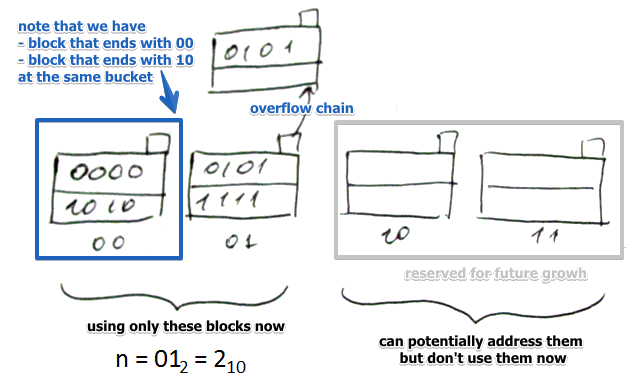• $b$ = 4 bits
• $i = 2$, can address $2^i = 4$ buckets
• $n = 01_2 = 2_{10}$ i.e. we use only two buckets at the moment
• the remaining 2 buckets are reserved for future growth
• note that in the first bucket there are 2 values: 0000 (2 last bits are $00$) and 1010 (with $10$)
• they are different but ended up in the same bucket
• since $10_2 \leqslant n = 01_2$ we flip the first significant bit of $10$ and get $00$
• also we allow overflow blocks for this data structure

## Increasing Parameters

### Increasing $n$

• when we increase $n$ we start using a new block
• and we need to re-organize data so the #Lookup Rule invariant is maintained
• if there's an overflow block, we will reduce it

Reorganization

• for that we see the block with current $n$ but most significant bit flipped
• i.e. for $10$ it's $00$
• then we go through all records there and move those that should belong to new block $10$
• after doing that the Lookup Rule invariant will be maintained

### Increasing $i$

If we increase $i$

• now number of buckets we can address becomes two times higher
• nothing will move: we don't touch $n$
• but $i$ gets increased only when $n$ increases, but doesn't fit to current $i$

### When

When it's better to increment $n$?

• Similar to ideas from Open Hashing Index
• $u = \cfrac{\text{# records}}{\text{# buckets}}$ where $u$ is space utilization
• and we set some threshold - once we exceed it, we increment $n$
• $i$ is incremented when $n$ becomes high enough so it no longer fits in $i$ bites

### Algorithm

When increasing $n$

• $n \leftarrow n + 1$
• if $n > i$, then $i \leftarrow i + 1$
• let $k$ be equal to $n$ with first significant bit flipped
• look for all keys that end with $n$ in the bucket #$k$
• and move them to the new bucket
• remove overflow blocks when needed

### Example

$b = 4$

Growing $n$

• suppose we have 2 blocks not in use, $n = 01$, $i = 2$
• we increase $n$: $n \leftarrow 10$
•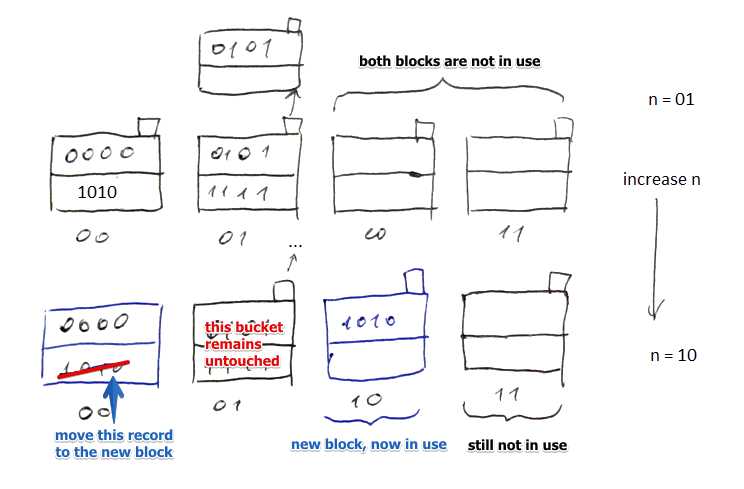• we transfer one record from 00 to 10 (which now becomes in use)

Removing overflow blocks

• suppose now we increase $n$ again: $n \leftarrow 11$
•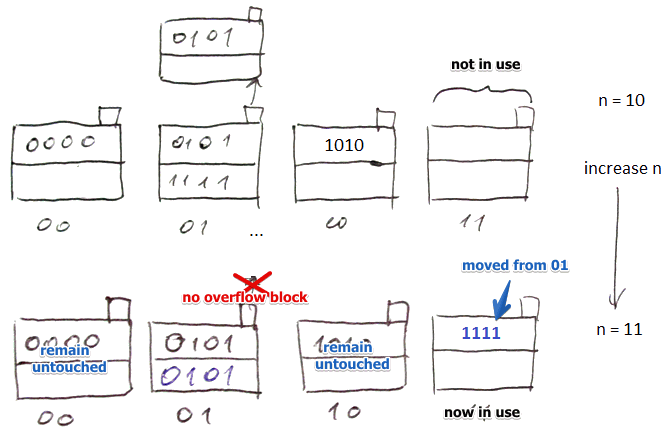• we transfer records from 01 to 11
• we move record 1111 to the new block
• since now there's some free room, we move records from the overflow block
• after moving them the overflow block becomes empty - so we may remove it altogether

note that in all cases we need to reorganize at most one bucket

Increasing $i$

• nothing moves as long as we don't touch $n$
• just append zeros before old bucket numbers + add new ones
•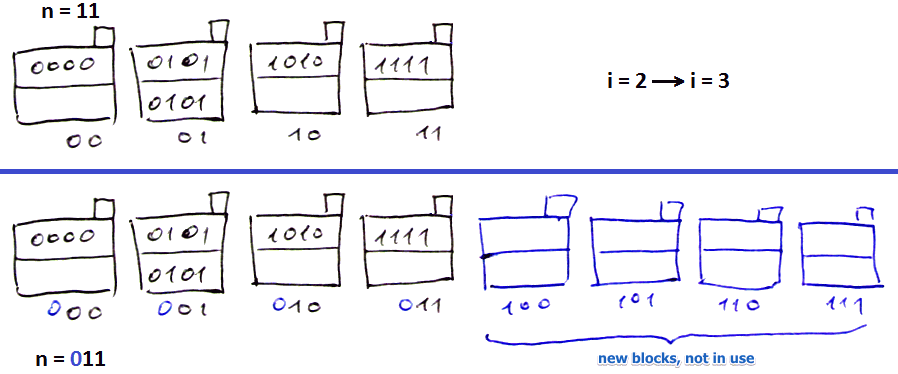• now can increase $n$ again

## Summary

• Can handle growing files (+)
• No additional level of indirection like in Extensible Hashing (+)
• Can still have overflow chains (-)

•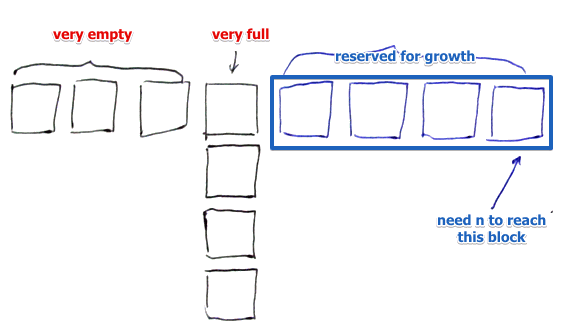• suppose for block $011$ we have huge overflow chain
• to reconstruct this chain, $n$ has to reach $111$ (twice more!)
• lots of time! especially when $i$ becomes longer